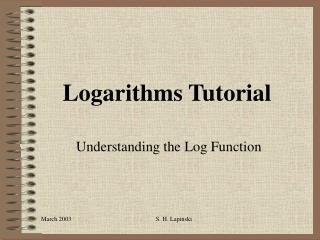# Logarithms Tutorial - PowerPoint PPT PresentationDownload PresentationLogarithms Tutorial

Logarithms Tutorial
Download Presentation## Logarithms Tutorial

- - - - - - - - - - - - - - - - - - - - - - - - - - - E N D - - - - - - - - - - - - - - - - - - - - - - - - - - -
##### Presentation Transcript

1. Logarithms Tutorial Understanding the Log Function S. H. Lapinski

2. Where Did Logs Come From? • The invention of logs in the early 1600s fueled the scientific revolution. Back then scientists, astronomers especially, used to spend huge amounts of time crunching numbers on paper. • By cutting the time they spent doing arithmetic, logarithms effectively gave them a longer productive life. • The slide rule was nothing more than a device built for doing various computations quickly, using logarithms.

3. There are still good reasons for studying them. • To model many natural processes, particularly in living systems. We perceive loudness of sound as the logarithm of the actual sound intensity, and dB (decibels) are a logarithmic scale. • To measure the pH or acidity of a chemical solution. • To measure earthquake intensity on the Richter scale.

4. How they are developed • In the mathematical operation of addition we take two numbers and join them to create a third 4 + 4 = 8 • We can repeat this operation: 4 + 4 + 4 = 12 • Multiplication is the mathematical operation that extends this: 3 • 4 = 12 • In the same way, we can repeat multiplication: 3 • 3 • 3 = 27 • The extension of multiplication is exponentiation: 3 • 3 • 3 = 27 = 33

5. More on development • The exponential function y = 2x is shown in this graph:

6. More on development • Now consider that we have a number and we want to know how many 2's must be multiplied together to get that number. • For example, given that we are using `2' as the base, how many 2's must be multiplied together to get 32? That is, we want to solve this equation: 2B = 32 • Of course, 25 = 32, so B = 5. To be able to get a hold of this, mathematicians made up a new function called the logarithm: log2 32 = 5

7. Inverses • This graph was created by switching the x and y of the exponential graph, which is the same as flipping the curve over on a 45 degree line.

8. DEFINITION: • The base a logarithm functiony = logax is the inverse of the base a exponential function y = ax (a > 0, a 1)

9. How to Convert Between Different Bases • Calculators and computers generally don't calculate the logarithm to the base 2, but we can use a method to make this easy. • Take for example, the equation 2x = 32. We use the change of base formula!!  We can change any base to a different base any time we want.  The most used bases are obviously base 10 and base e because they are the only bases that appear on your calculator! Pick a new base and the formula says it is equal to the log of the number in the new base divided by the log of the old base in the new base.

10. Examples • Find the value oflog2 37 • Change to base 10 and use your calculator. log 37/log 2 • Now use your calculator and round to hundredths. = 5.21 • Log7 99 = ? • Change to base 10 or base e.  Try it both ways and see. • log3 81 • log4 256 • log2 1024

11. Properties Of Logarithms • For any real numbers x > 0 and y >0, •  Product Rule: loga xy = loga x + loga y •  Quotient Rule: •  Power Rule: loga xy = yloga x

12. More Practice • Express each as a single log. • Log x + Log y - Log z = • 2 Ln x + 3 Ln y = • Solve • Log2 (x + 1) + Log2 3 = 4 • Log (x + 3) + Log x = 1

13. Web Sites • http://www.shodor.org/UNChem/math/logs/ • http://www.physics.uoguelph.ca/tutorials/LOG/ • http://www.purplemath.com/modules/logs.htm • http://www.exploremath.com/activities/Activity_page.cfm?ActivityID=7 • SAMPLE Test on Logs • http://www.alltel.net/~okrebs/page58.html